# Vibrating String

The fundamental vibrational mode of a stretched string is such that the wavelength is twice the length of the string.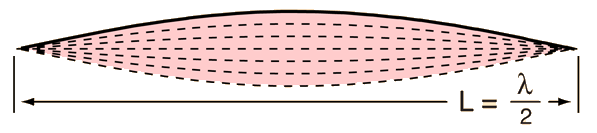Applying the basic wave relationship gives an expression for the fundamental frequency: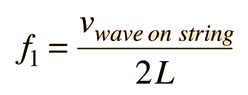### Calculation

 Since the wave velocity is given by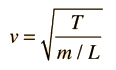, the frequency expression

can be put in the form: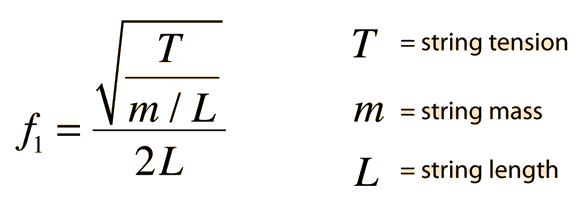The string will also vibrate at all harmonics of the fundamental. Each of these harmonics will form a standing wave on the string.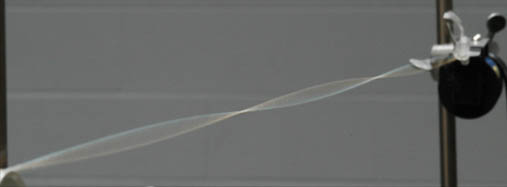This shows a resonant standing wave on a string. It is driven by a vibrator at 120 Hz.For strings of finite stiffness, the harmonic frequencies will depart progressively from the mathematical harmonics. To get the necessary mass for the strings of an electric bass as shown above, wire is wound around a solid core wire. This allows the addition of mass without producing excessive stiffness.

 Example measurements on a steel string
 String frequencies String instruments Illustration with a slinky Mathematical form
Index

Periodic motion concepts

Resonance concepts

 HyperPhysics***** Sound R Nave
Go Back

# Wave Velocity in String

The velocity of a traveling wave in a stretched string is determined by the tension and the mass per unit length of the string.

The wave velocity is given byShow

When the wave relationship is applied to a stretched string, it is seen that resonant standing wave modes are produced. The lowest frequency mode for a stretched string is called the fundamental, and its frequency is given by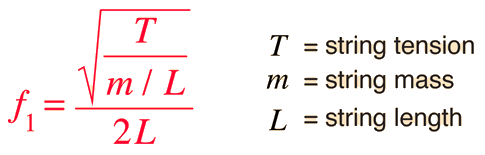From

velocity = sqrt ( tension / mass per unit length )

the velocity = m/s
when the tension = N = lb
for a string of length cm and mass/length = gm/m.
For such a string, the fundamental frequency would be Hz.

Any of the highlighted quantities can be calculated by clicking on them. If numerical values are not entered for any quantity, it will default to a string of 100 cm length tuned to 440 Hz. Default values will be entered for any quantity which has a zero value. Any quantities may be changed, but you must then click on the quantity you wish to calculate to reconcile the changes.

 Derivation of wave speed
Index

Periodic motion concepts

Resonance concepts

 HyperPhysics***** Sound R Nave
Go Back

# Harmonics

 An ideal vibrating string will vibrate with its fundamental frequency and all harmonics of that frequency. The position of nodes and antinodes is just the opposite of those for an open air column. The fundamental frequency can be calculated fromwhere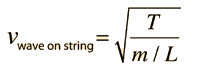T = string tensionm = string massL = string length and the harmonics are integer multiples.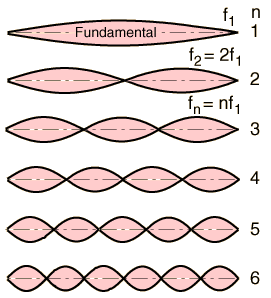Index

Periodic motion concepts

Resonance concepts

 HyperPhysics***** Sound R Nave
Go Back

# Vibrating String Frequencies

If you pluck your guitar string, you don't have to tell it what pitch to produce - it knows! That is, its pitch is its resonant frequency, which is determined by the length, mass, and tension of the string. The pitch varies in different ways with these different parameters, as illustrated by the examples below:

 If you have a string with starting pitch: 100 Hz and change* to the pitch will be double the length 50 Hz four times the tension 200 Hz four times the mass 50 Hz
*with the other parameters reset to their
original values.
If you want to raise the pitch of a string by increasing its tension:
 Tension Frequency Original T0 f0 1 octave up 4T0 2f0 2 octaves up 16T0 4f0 3 octaves up 64T0 8f0 4 octaves up 256T0 16f0 5 octaves up 1024T0 32f0

You can see that it is not practical to tune a string over a large pitch range using the tension, since the tension goes up by the square of the pitch ratio.

 Calculation
Index

Periodic motion concepts

Resonance concepts

 HyperPhysics***** Sound R Nave
Go Back